You may also like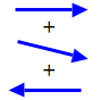Arrow Arithmetic 1

The first part of an investigation into how to represent numbers using geometric transformations that ultimately leads us to discover numbers not on the number line.Arrow Arithmetic 2

Introduces the idea of a twizzle to represent number and asks how one can use this representation to add and subtract geometrically.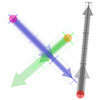Arrow Arithmetic 3

How can you use twizzles to multiply and divide?

Twizzle Arithmetic

Age 14 to 16 Challenge Level:

In Arrow Arithmetic 2 and Arrow Arithmetic 3 (published in February 2007), the problems were about finding a way to do arithmetic using geometry where numbers are represented by a double arrow. You might need to refer back to these problems to remind yourself of the idea.

Now take a look at these four audio/visual clips to see the four arithmetic operations in action: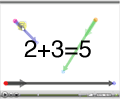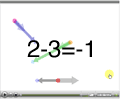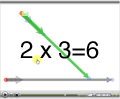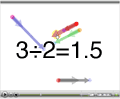And, in case you'd prefer it in text, here's a summary of the geometrical technique:
 Addition Make all the unit arrows agree in length and direction. Then arrange the number arrows in a nose-to-tail chain. The answer is represented by a number arrow that stretches from the tail of the first arrow in the chain to the head of the last arrow in the chain. Subtraction Rotate the number arrow of the the number to subtract by a half turn with respect to its unit arrow. Then add. Multiplication Rotate, translate, and scale until the unit arrow of one number is the same as the number arrow of the other. Ignore these two equated arrows. The product is formed from the remaining number and unit arrows. Division Take the reciprocal of the divisor by swapping its number and unit arrows. Then multiply.

Try adding the green arrow-pair (which I'll call a twizzle ) to the blue twizzle using the addition rule. Here's an animation in which to experiment - you should find that the grey twizzle represents the answer..

Full Screen Version
If you can see this message Flash may not be working in your browser
Please see http://nrich.maths.org/techhelp/#flash to enable it.

Now let's try multiplication. In the next animation, the grey twizzle is the product of the blue twizzle and the green twizzle:
Full Screen Version
If you can see this message Flash may not be working in your browser
Please see http://nrich.maths.org/techhelp/#flash to enable it.

Check you can do the multiplication geometrically yourself.

Click on the twizzle spots and notice the names that these numbers have. These are shown when you roll the mouse over the number arrow. Each number has two names (click the spot again to see the second 'polar' name).

Notice that a number arrow of length r turned through an angle d degrees is called r cis (d) .
Notice that 1 cis (90) has the special name i .
What is the value of i 2 ?

Here's the problem :

Calculate (1+i)(1+i) geometrically and by multiplying out the brackets. Use Pythagoras' theorem to show that both methods give the same answer.